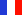###### Descriptions and Examples for the POV-Ray Raytracerby Friedrich A. Lohmüller Elementary Geometry for Raytracing
ItalianoFrançaisDeutschHome
- POV-Ray Tutorial

- Geometrical Basics
for Raytracing

Right-angled Triangle
Pythagorean Theorem
Trigonometry Basics
Law of cosines
Equilateral Triangle
Regular Polygon
Polyhedron
Tetrahedron
Octahedron
Cube & Cuboid
Dodecahedron
Icosahedron
Cuboctahedron
Truncated Octahedron
Rhombicuboctahedron
Truncated Icosahedron
Circles
Tangent circles
Internal Tangents
External Tangents

- Geometric 3D Animations
- Geometric Shapes
in POV-Ray
___________________

## This is a small collection of some useful geometrical basics. This does not intend to be complete!

 Right-angled Triangle - Pythagoras - Trigonometry Right-angled Triangle Pythagorean Theorem Trigonometry Basics Law of Cosines
 Regular Polygons Equilateral Triangle Regular Polygon
 Regular Polyhedron - the five Platonic solids Tetrahedron Octahedron Cube+Cuboid Dodecahedron Icosahedron Cuboctahedron TruncatedOctahedron Rhombicub-octahedron TruncatedIcosahedron
 Circles Tangent Circles Internal Tangents External Tangents

top

 © Friedrich A. Lohmüller, 2012 www.f-lohmueller.de Visitors: (since May-1999) Thanks for your visit!